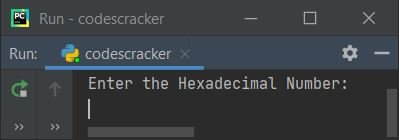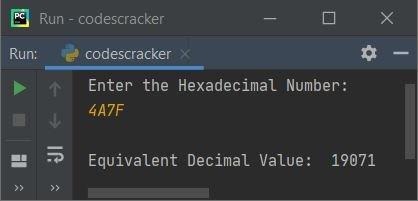# Python Program to Convert Hexadecimal to Decimal

In this article, I've created some programs in Python, to convert hexadecimal number entered by user at run-time to its equivalent decimal value. Here are the list of programs:

• Hexadecimal to Decimal using while Loop
• Using int() Method
• Using user-defined Function
• Using Class

Note - Before creating these programs, if you're not aware about steps used for the conversion, then refer to Hexadecimal to Decimal Steps, Formula, Example to get every required things about the topic.

## Hexadecimal to Decimal using while Loop

To convert hexadecimal to decimal number in Python, you have to ask from user to enter a hexadecimal number, then convert that number into its equivalent decimal value as shown in the program given below:

```print("Enter the Hexadecimal Number: ")
hexdecnum = input()

chk = 0
decnum = 0
hexdecnumlen = len(hexdecnum)
hexdecnumlen = hexdecnumlen-1
i = 0
while hexdecnumlen>=0:
rem = hexdecnum[hexdecnumlen]
if rem>='0' and rem<='9':
rem = int(rem)
elif rem>='A' and rem<='F':
rem = ord(rem)
rem = rem-55
elif rem>='a' and rem<='f':
rem = ord(rem)
rem = rem-87
else:
chk = 1
break
decnum = decnum + (rem * (16 ** i))
hexdecnumlen = hexdecnumlen-1
i = i+1

if chk == 0:
print("\nEquivalent Decimal Value: ", decnum)
else:
print("\nInvalid Input!")```

Here is its sample run:Now supply the input say 4A7F as hexadecimal number and press `ENTER` key to convert and print its equivalent decimal value as shown in the snapshot given below:Note - The len() method is used to find length of a string. That is, this method returns length of string passed as its argument.

Note - The ord() method is used to find Unicode of a character passed as its argument. For example, ord('A') returns 65, ord('B') returns 66, ord('c') returns 99 etc.

Note - The ** is used to calculate value of a number to any power. For example num ** i gets treated as numi. That is, 16 ** 3 returns the value of 163, which will be 16*16*16 or 4096

The dry run of above program with user input 4A7F goes like:

• Initial values, hexdecnum=4A7F (entered by user), chk=0, decnum=0, hexdecnumlen=4 (length of hexdecnum or 4A7F), i=0
• Since the value of hexdecnumlen is decremented by 1. Therefore hexdecnumlen=3
• Now the condition (of while loop) hexdecnumlen>=0 or 3>=0 evaluates to be true, therefore program flow goes inside the loop
• hexdecnum[hexdecnumlen] or hexdecnum or 'F' (last index's character of hexdecnum) gets initialized to rem
• Since rem's value is 'F', therefore the the condition of first elif (that is rem>='A' and rem<='F') evaluates to be true, therefore program flow goes inside its body and ord(rem) or ord('F') or 70 gets initialized to rem. And using the second statement, rem-55 or 70-55 or 15 (15 corresponds to 'F' in hexadecimal number system) initialized to rem
• Now decnum + (rem * (16 ** i)) or 0 + (15 * (160)) or 15 * 1 or 15 gets initialized to decnum
• hexdecnumlen-1 or 3-1 or 2 gets initialized to hexdecnumlen
• i+1 or 0+1 or 1 gets initialized to i
• Now program flow goes back and evaluates the condition of while loop again with new value of hexdecnumlen
• That is, the condition hexdecnumlen>=0 or 2>=0 evaluates to be true again, therefore program flow goes inside the loop. This process continues until the condition evaluates to be false
• In this way, the given number (in hexadecimal) gets converted into its equivalent decimal value
• So after exiting from the loop, print the value of decnum, if chk's value is equal to 0 (initial value)
• And if chk's value is not equal to 0 (its initial value), means that program flow goes inside the else's body once. And that means, an invalid hex digit was entered by user

#### Modified Version of Previous Program

This program uses end= to skip printing of an automatic newline using print()

```print("Enter Hexadecimal Number: ", end="")
hnum = input()

chk = dnum = i = 0
hlen = len(hnum) - 1
while hlen>=0:
if hnum[hlen]>='0' and hnum[hlen]<='9':
rem = int(hnum[hlen])
elif hnum[hlen]>='A' and hnum[hlen]<='F':
rem = ord(hnum[hlen]) - 55
elif hnum[hlen]>='a' and hnum[hlen]<='f':
rem = ord(hnum[hlen]) - 87
else:
chk = 1
break
dnum = dnum + (rem * (16 ** i))
hlen = hlen - 1
i = i+1

if chk == 0:
print("\nEquivalent Decimal Value = ", dnum)
else:
print("\nInvalid Input!")```

Here is its sample run with hexadecimal input as 23D5:## Hexadecimal to Decimal using int()

This program uses int() method to do the same job. The int() method returns integer (decimal) equivalent of any value passed as its argument.

```print("Enter Hexadecimal Number: ", end="")
hnum = input()

dnum = int(hnum, 16)
print("\nEquivalent Decimal Value =", dnum)```

## Hexadecimal to Decimal using Function

This program is created using user-defined and predefined functions. The user-defined function used here is HexToDec(). This function takes a value (hexadecimal) as its argument and returns its decimal equivalent using int() method:

```def HexToDec(h):
return int(h, 16)

hnum = input()

dnum = HexToDec(hnum)
print("\nEquivalent Decimal Value =", dnum)```

## Hexadecimal to Decimal using Class

This is the last program of this article, created using a class named CodesCracker. Class is an object-oriented feature of Python language.

```class CodesCracker:
def HexToDec(self, h):
return int(h, 16)

hnum = input()

ob = CodesCracker()
dnum = ob.HexToDec(hnum)
print("\nEquivalent Decimal Value =", dnum)```

Since to access any member function of a class in Python, we must have to create an object of that class. Therefore, an object named ob of class CodesCracker is created. And using this object, we've accessed the member function named HexToDec() using dot (.) operator.

#### Same Program in Other Languages

Python Online Test

« Previous Program Next Program »